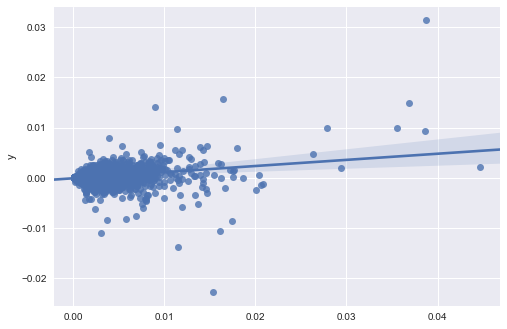# G-Research Financial Forecasting Challenge¶

## Tutorial¶

The aim of this tutorial is to load the training data set, fit some simple models, and generate a submission file.

First, load some data science libraries

In :
%matplotlib inline
import numpy as np
import pandas as pd
import matplotlib.pyplot as plt
import seaborn as sns
import sklearn.linear_model


Read the training data set and display the first few rows.

In :
df = pd.read_csv('train.csv', index_col=0)

Out:
Market Day Stock x0 x1 x2 x3A x3B x3C x3D x3E x4 x5 x6 y Weight
Index
0 1 1 363 0.002696 0.530040 0.400810 1.300048e-06 4.639533e-06 1.073594e-05 2.180516e-05 4.416943e-05 0.002212 6.214737e-07 200.000000 -0.000196 1.533780
1 1 1 1223 0.081093 1.366218 1.325157 1.656861e-05 3.024668e-05 5.261872e-05 4.193762e-05 4.193762e-05 0.010932 3.414868e-05 300.000000 -0.003798 0.843604
2 1 1 1372 0.009561 0.464871 0.328008 6.500872e-08 2.323204e-07 5.182686e-07 1.080834e-06 2.030442e-06 0.000306 4.079460e-08 159.692076 -0.000003 29.824024
3 1 1 1942 0.045300 0.608222 0.456662 1.928694e-08 6.495275e-08 1.338509e-07 2.644214e-07 6.980798e-07 0.000205 9.502066e-09 212.160378 -0.000008 100.388668
4 1 1 2712 0.001605 0.476718 0.205069 6.759866e-07 2.638783e-06 6.424519e-06 1.189923e-05 2.064450e-05 0.001525 3.428403e-07 153.724351 0.000065 5.950714

Now read the test data set. Note that the return $y$ and weights $w$ are missing

In :
df_test = pd.read_csv('test.csv', index_col=0)

Out:
Market Day Stock x0 x1 x2 x3A x3B x3C x3D x3E x4 x5 x6
Index
0 1 13 363 0.000842 0.436594 0.477848 1.535381e-06 4.353950e-06 7.718616e-06 1.632886e-05 3.267851e-05 0.001661 9.724611e-07 112.968475
1 1 13 1223 0.020800 0.636143 0.670798 5.204133e-06 9.546012e-06 1.575258e-05 2.804666e-05 1.082500e-05 0.002104 2.146299e-05 100.000000
2 1 13 1372 0.068580 0.478891 0.481816 5.433730e-08 1.733539e-07 3.866963e-07 8.520664e-07 2.103176e-06 0.000222 3.085296e-08 188.335508
3 1 13 1942 0.011986 0.463201 0.294900 1.103215e-08 3.555558e-08 7.280376e-08 1.364231e-07 3.155050e-07 0.000141 1.678216e-08 212.123913
4 1 13 2712 0.000164 0.549980 0.352332 4.494670e-07 8.503405e-07 1.222847e-06 1.901114e-06 4.048304e-06 0.001235 4.595200e-07 124.024977

## 1. Mean model¶

Firstly, we will try a simple model that the predicted return is equal to the mean return for each stock.

In :
stock_mean = df.groupby('Stock')['y'].mean()

Out:
Stock
0    0.000890
1    0.000151
2   -0.000069
3   -0.000109
4    0.000023
Name: y, dtype: float64

Now we use the stockwise means to make predictions $y_p$ for the test set and write them to a csv file.

In :
yp = df_test['Stock'].map(stock_mean).rename('y')

Out:
Index
0    0.000482
1   -0.000071
2    0.000025
3    0.000019
4    0.000112
Name: y, dtype: float64

Unfortunately, one of the values is not a number (NaN). We could try replacing this with a prediction of 0.

In :
yp[yp.isnull()]

Out:
Index
43289   NaN
Name: y, dtype: float64
In :
yp.fillna(0).to_csv('mean_model.csv', header=True)


## 2. Linear model¶

Choose one of the predictors $x_4$ and build a linear model of the form $$y_p = \alpha + \beta x_4$$

In :
model = sklearn.linear_model.LinearRegression()

X = df[['x4']]
y = df['y']
model.fit(X, y)

print('alpha:  \t', model.intercept_)
print('beta:   \t', model.coef_)

print('r^2 score:\t', model.score(X, y))

alpha:  	 -7.28920282437e-05
beta:   	 0.0836916860756
r^2 score:	 0.0341790057712


Make a plot using seaborn (only plot every 100th point to save time)

In :
sns.regplot(X[::100], y[::100]);Use the model to make predictions and write them to csv

In :
yp = pd.Series(model.predict(df_test[['x4']])).rename('y')
yp.index.name = 'Index'

Out:
Index
0    0.000066
1    0.000103
2   -0.000054
3   -0.000061
4    0.000030
Name: y, dtype: float64
In :
yp.to_csv('linear_model.csv', header=True)

In [ ]: